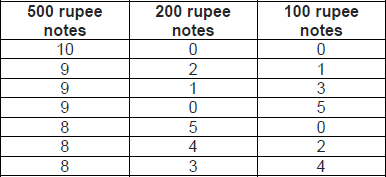### CAT 2019 - Slot 1 - Logical Reasoning and Data Interpretation - An ATM dispenses exactly Rs. 5000 per withdrawal

##### An ATM dispenses exactly Rs. 5000 per withdrawal using 100, 200 and 500 rupee notes. The ATM requires every customer to give her preference for one of the three denominations of notes. It then dispenses notes such that the number of notes of the customer’s preferred denomination exceeds the total number of notes of other denominations dispensed to her.

Q. 1:In how many different ways can the ATM serve a customer who gives 500 rupee notes as her preference?

##### Q. 2: If the ATM could serve only 10 customers with a stock of fifty 500 rupee notes and a sufficient number of notes of other denominations, what is the maximum number of customers among these 10 who could have given 500 rupee notes as their preferences?

Q. 3: What is the maximum number of customers that the ATM can serve with a stock of fifty 500 rupee notes and a sufficient number of notes of other denominations, if all the customers are to be served with at most 20 notes per withdrawal?
1. 10
2. 16
3. 12
4. 13

Q. 4: What is the number of 500 rupee notes required to serve 50 customers with 500 rupee notes as their preferences and another 50 customers with 100 rupee notes as their preferences, if the total number of notes to be dispensed is the smallest possible?
1. 800
2. 750
3. 900
4. 1400

The ATM dispenses only 500, 200 and 100 notes and since 500 rupee notes is the preference, it has to dispense more 500 rupee notes than the other two notes combined. The following ways are possible:Hence, a total of seven ways are possible. Ans : 7

Question 2:

To serve the maximum number of customers with 500 rupee notes as preference, we need to minimize the number of 500 rupee notes that can be served to any person.

From the above solution, the minimum number of 500 rupee notes that the ATM can dispense to any person with 500 rupee notes as his/her preference is 8. Hence, with fifty 500 rupee notes, a total of 6 persons can be served. Ans : 6

Question 3:

Since there are a limited number of 500 rupee notes, we can minimize the number of 500 rupee notes dispensed to each customer, while ensuring that each customer is served at most 20 notes.

If no 500 rupee notes is dispensed, the minimum number of notes that must be dispensed is 25 (all 200 rupee notes). This is not possible.

If one 500 rupee note is dispensed, the minimum number of notes is 14 (one 500 rupee note, twelve 200 rupee notes and one 100 rupee note). This is also not possible.

If two 500 rupee notes are dispensed, the minimum number of notes is 22 (two 500 rupee notes and twenty 200 rupee notes).

If three 500 rupee notes are dispensed, the minimum number of notes is 21 (three 500 rupee notes, seventeen 200 rupee notes and one 100 rupee note). If four 500 rupee notes are dispensed, the minimum number of notes is 19 (four 500 rupee notes and fifteen 200 rupee notes). Hence, the minimum number of 500 rupee notes that can be dispensed to any person is 4. With fifty 500 rupee notes, a maximum of 12 persons can be served. Ans : 12

Question 4:

To dispense the smallest possible number of notes to a person with 500 rupee notes as his/her preference, the ATM should dispense all 500 rupee notes. Hence, minimum number of notes required to serve any person with 500 rupee notes as his/her preference = 10 (all of 500 rupees).

Total number of 500 rupee notes required to serve 50 customers with 500 rupee notes as his/her preference = 10 × 50 = 500

To minimize the number of notes to be served to a person with 100 rupee notes as his/her preference, we can maximize the number of 500 rupee notes served to him, keeping the 100 rupee notes more than the sum of the other two denominations.

This is possible if the machine serves eight 500 rupee notes and ten 100 rupee notes. Hence, the total number of 500 rupee notes required to serve 50 customers with 100 rupee notes as his/her preference = 8 × 50 = 400

Total number of 500 rupee notes required in the given scenario = 500 + 400 = 900 Ans : 900

Note: Given that the ATM dispenses 500, 200 and 100 rupee notes. A possible interpretation of this is that at least one note of each denomination is dispensed. However, as there is no additional information supporting this, you should also consider the cases in which not all the three denominations are dispensed.

Inspiring Education… Assuring Success!!

Ghatkopar | Borivali | Andheri | Online